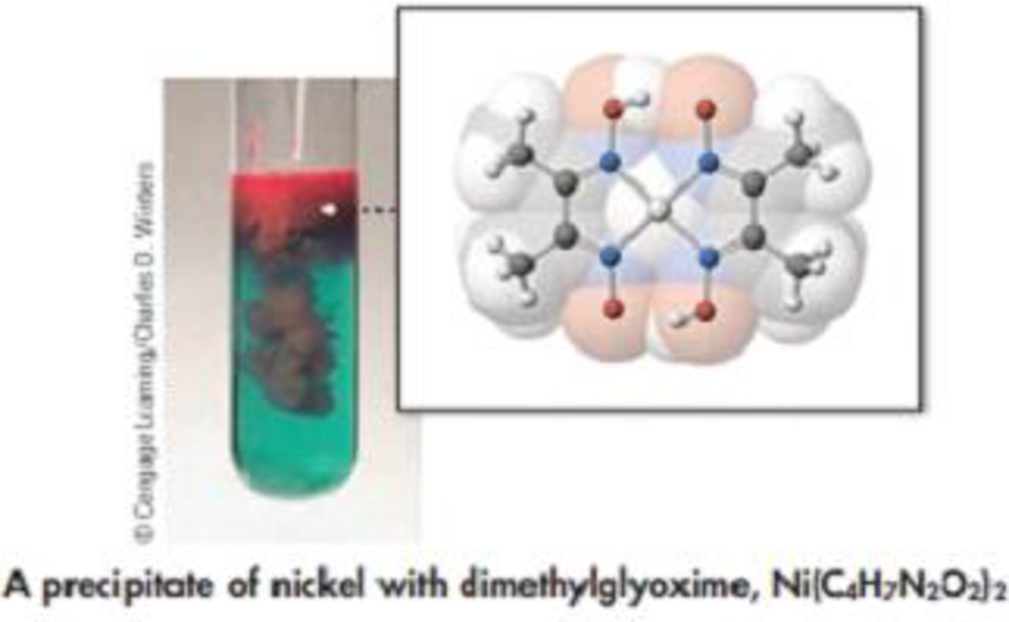Chapter 4, Problem 29PS

Chapter
Section
Textbook Problem

Nickel(II) sulfide, NiS, occurs naturally as the relatively rare mineral millerite. One of its occurrences is in meteorites. To analyze a mineral sample for the quantity of NiS, the sample is dissolved in nitric add to form a solution of Ni(NO3)2.NiS(s) + 4 HNO3(aq) → Ni(NO3)2(aq) + 2 NO2(g) + 2 H2O(l) + S(s)The aqueous solution of Ni(NO3)2 is then reacted with the organic compound dimethylglyoxime (C4H8N2O2) to give the red solid Ni((C4H7N2O2)2.Ni(NO3)2(aq) + 2 (C4H8N2O2)(aq) → Ni(C4H7N2O2)2 + 2 HNO3(aq)Suppose a 0.468-g sample containing millerite produces 0.206 g of red, solid Ni(C4H7N2O2)2What is the mass percent of NiS in the sample?Interpretation Introduction

Interpretation:

The mass percent of NiS in the given sample has to be determined.

Concept introduction:

• The relation between the number of moles and mass of the substance is ,

Numberofmole=MassingramMolarmass

Massingramofthesubstance=Numberofmole×Molarmass

• The molar mass of an element or compound is the mass in grams of 1 mole of that substance, and it is expressed in the unit of grams per mol (g/mol).
• For chemical reaction balanced chemical reaction equation written in accordance with the Law of conservation of mass.
• Law of conservation of mass states that for a reaction total mass of the reactant and product must be equal.
• Stoichiometric factor is a relationship between reactant and product which is obtained from the balanced chemical equation for a particular reaction.
• Amountof substance=Concentrationofsubstance×volumeofthesubstance
• Mass percent of elements of a compound is the ratio of mass of element to the mass of whole compound and multiplied with hundred.
Explanation

Herein to analyze a mineral sample for the quantity of NiS, the sample is dissolved in nitric acid to form a solution of Ni(NO3)2

The balanced equation for this reaction is,

NiS(s)+4HNO3(aq)Ni(NO3)2(aq)+2NO3(g)+2H2O(l)+S(s)

The aqueous solution of Ni(NO3)2 is then reacted with the organic compound dimethylglyoxime C4H8N2O2 to give the red solid Ni(C4H7N2O2)2

Ni(NO3)2(aq)+2C4H8N2O2(aq)Ni(C4H8N2O2)2(s)+2HNO3(aq)

From the amount of Ni(C4H8N2O2)2 involved in the reaction, amount of NiS can be calculated

Still sussing out bartleby?

Check out a sample textbook solution.

See a sample solution

The Solution to Your Study Problems

Bartleby provides explanations to thousands of textbook problems written by our experts, many with advanced degrees!

Get Started

The best and safest source of vitamin D for people in the United States is exposure to sunshine. T F

Nutrition: Concepts and Controversies - Standalone book (MindTap Course List)

4. The normal arterial range is

Cardiopulmonary Anatomy & Physiology

Write the molecular formula for each alkane.

Introduction to General, Organic and Biochemistry